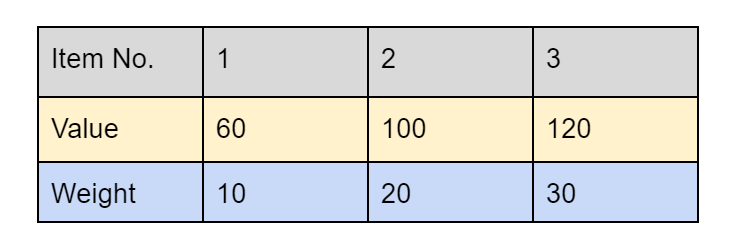# Fractional Knapsack Problem : Greedy Approach

Problem Statement: The weight of N items and their corresponding values are given. We have to put these items in a knapsack of weight W such that the total value obtained is maximized.

Note: We can either take the item as a whole or break it into smaller units.

```Example:

Input: N = 3, W = 50, values[] = {100,60,120}, weight[] = {20,10,30}.

Output: 240.00

Explanation: The first and second items  are taken as a whole  while only 20 units of the third item is taken. Total value = 100 + 60 + 80 = 240.00
```

### Solution

Disclaimer: Don’t jump directly to the solution, try it out yourself first.

Approach

The greedy method to maximize our answer will be to pick up the items with higher values. Since it is possible to break the items as well we should focus on picking up items having higher value /weight first. To achieve this, items should be sorted in decreasing order with respect to their value /weight. Once the items are sorted we can iterate. Pick up items with weight lesser than or equal to the current capacity of the knapsack. In the end, if the weight of an item becomes more than what we can carry, break the item into smaller units. Calculate its value according to our current capacity and add this new value to our answer.

Let’s understand with an example:-

N = 3, W = 50, values[] = {100,60,120}, weight[] = {20,10,30}.

The value/weight of item 1 is (100/20) = 5,for item 2 is (60/10) = 6 and for item 3 is (120/30) = 4.

Sorting them in decreasing order of value/weight we haveInitially capacity of bag(W)  = 50, value = 0

Item 1 has a weight of 10, we can pick it up.

Current weight = 50 – 10 = 40 ,Current value = 60.00

Item 2  has a weight of 20 , we can pick it up.

Current weight = 40 – 20 = 20 ,Current value = 60.00 + 100.00 = 160.00

Item 3 has a weight of 30 , but current knapsack capacity is 20.Only a fraction of it is chosen.

Current weight = 20 – 20 = 0 ,Final value = 160.00 + (120/30 )*20 = 240.00

Code:

## C++ Code

``````#include <bits/stdc++.h>

using namespace std;

struct Item {
int value;
int weight;
};
class Solution {
public:
bool static comp(Item a, Item b) {
double r1 = (double) a.value / (double) a.weight;
double r2 = (double) b.value / (double) b.weight;
return r1 > r2;
}
// function to return fractionalweights
double fractionalKnapsack(int W, Item arr[], int n) {

sort(arr, arr + n, comp);

int curWeight = 0;
double finalvalue = 0.0;

for (int i = 0; i < n; i++) {

if (curWeight + arr[i].weight <= W) {
curWeight += arr[i].weight;
finalvalue += arr[i].value;
} else {
int remain = W - curWeight;
finalvalue += (arr[i].value / (double) arr[i].weight) * (double) remain;
break;
}
}

return finalvalue;

}
};
int main() {
int n = 3, weight = 50;
Item arr[n] = { {100,20},{60,10},{120,30} };
Solution obj;
double ans = obj.fractionalKnapsack(weight, arr, n);
cout << "The maximum value is " << setprecision(2) << fixed << ans;
return 0;
}
``````

Output:

The maximum value is 240.00

Time Complexity: O(n log n + n). O(n log n) to sort the items and O(n) to iterate through all the items for calculating the answer.

Space Complexity: O(1), no additional data structure has been used.

## Java Code

``````import java.util.*;
class Item {
int value, weight;
Item(int x, int y) {
this.value = x;
this.weight = y;
}
}

class itemComparator implements Comparator<Item>
{
@Override
public int compare(Item a, Item b)
{
double r1 = (double)(a.value) / (double)(a.weight);
double r2 = (double)(b.value) / (double)(b.weight);
if(r1 < r2) return 1;
else if(r1 > r2) return -1;
else return 0;
}
}
public class solve{
static double fractionalKnapsack(int W, Item arr[], int n) {
Arrays.sort(arr, new itemComparator());

int curWeight = 0;
double finalvalue = 0.0;

for (int i = 0; i < n; i++) {

if (curWeight + arr[i].weight <= W) {
curWeight += arr[i].weight;
finalvalue += arr[i].value;
}

else {
int remain = W - curWeight;
finalvalue += ((double)arr[i].value / (double)arr[i].weight) * (double)remain;
break;
}
}

return finalvalue;

}
public static void main(String args[])
{
int n = 3, weight = 50;
Item arr[] = {new Item (100,20),new Item(60,10),new Item(120,30)};
double ans = fractionalKnapsack(weight, arr, n);
System.out.println("The maximum value is "+ans);
}
}``````

Output:

The maximum value is 240.00

Time Complexity: O(n log n + n). O(n log n) to sort the items and O(n) to iterate through all the items for calculating the answer.

Space Complexity: O(1), no additional data structure has been used.

Special thanks to Somparna Chakrabarti for contributing to this article on takeUforward. If you also wish to share your knowledge with the takeUforward fam, please check out this article